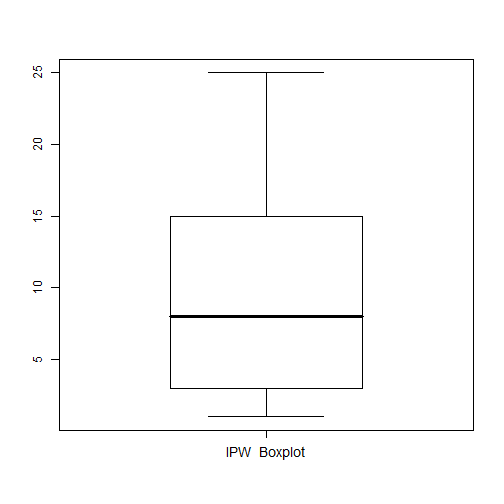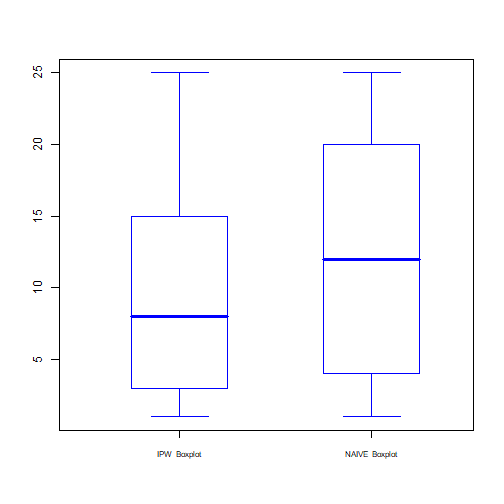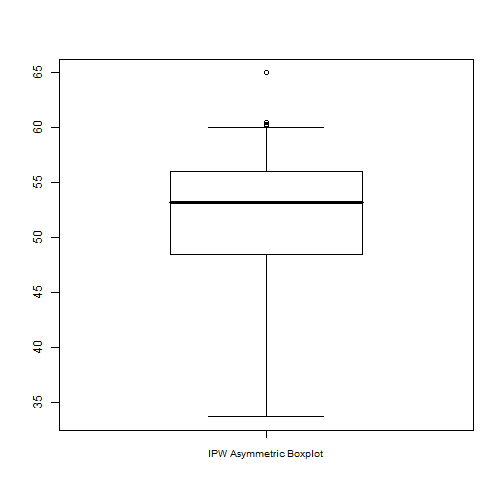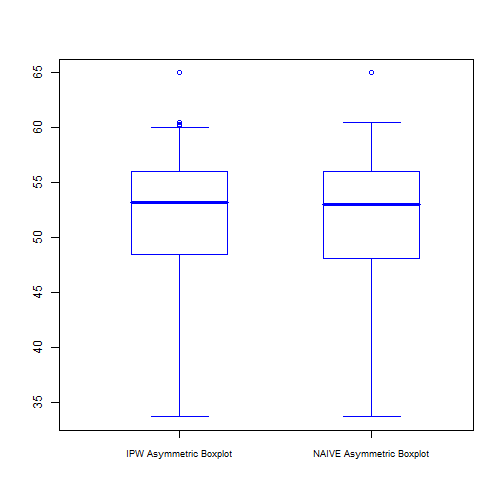# Introduction

IPWboxplot is a contributed R package for drawing boxplots adapted to the happenstance of missing observations when drop-out probabilities are given by the practitioner or modelled using auxiliary covariates. It also provides a function to estimate asymptotically unbiased quantiles based on inverse probability weighting (IPW) as in Zhang et al. (2012). For that purpose, a missing at random model is assumed. These IPW quantiles are used to compute the measures needed to construct the boxplot and hence, to calculate the outlier cut–off values.

This document gives a quick tour of IPWboxplot (version 0.1.1) functionalities. It was written in R Markdown, using the knitr package for production. See help (package="IPWboxplot") for further details and references provided by citation ("IPWboxplot").

library(IPWboxplot)


# Inverse Probability Weighted Quantiles

The function IPW.quantile computes the IPW quantiles of a vector y containing missing observations when auxiliary information from a vector of drop-out probabilities supplied by the user or from a set of covariates is available. The dataset boys of the R package mice allows us to illustrate the use of this function.

The dataset contains 748 observations and the variable y=tv has 522 missing observations. For illustrative purposes, we consider the variable age, which is completely observed, as covariate with predictive capability for the propensity. By default, a logistic model is used to fit the happenstance probabilities. The following code returns the $\alpha-$quantiles corresponding to $$\alpha=$$ 0.25, 0.5, 0.75 and 0.9 of the variable “Testicular volume (tv)” using inverse probability weighting.

library(mice)
#> Warning: package 'mice' was built under R version 4.1.3
data(boys)
attach(boys)
dim(boys)
#>  748   9
res=IPW.quantile(tv,x=age,probs=c(0.25,0.5,0.75,0.9))
ls(res)
#>  "IPW.quantile" "px"
#res$px is the vector of estimated drop-out probabilities #res$IPW.quantile is the vector of estimated IPW quantiles
res$IPW.quantile #>  3 8 15 20  # Inverse Probability Weighted Boxplot The function IPW.boxplot draws the modified boxplot adapted to missing data using the IPW quantiles. The function also returns a list of statistical summaries. As default, the function returns only the adapted boxplot and the statistics computed by inverse probability weighting. res=IPW.boxplot(tv,x=age,main=" ")#> The method used to estimate the dropout probability is LOGISTIC #> IPW Quartiles #> 25% 50% 75% #> 3 8 15 #> Lower and upper whiskers of the IPW Boxplot #> Lower Upper #> 1 25  The function returns a list containing the quartiles, the lower and upper whiskers of the IPW boxplot, the observations considered as outliers and the vector of estimated or given drop-out probabilities. ls(res) #>  "IPW.Quartiles" "IPW.whisker" "out.IPW" "px"  As shown in Figure 1, the IPW boxplot does not detect ouliers for this data set. res$out.IPW
#> integer(0)


Specifying both in the argument “graph”, the function allows to compare the adapted boxplot with the naive boxplot obtained by simply dropping out the missing observations. In this situation, besides the measures related to the IPW boxplot, the function also returns the quartiles, whiskers and detected outliers obtained with the observations at hand which are associated to naive boxplot.

res1=IPW.boxplot(tv,x=age,graph="both",color="blue",size.letter=0.7,main=" ")#> The method used to estimate the dropout probability is  LOGISTIC
#> IPW Quartiles
#>  25%    50%   75%
#>  3 8 15
#> Naive Quartiles
#>  25%    50%   75%
#>  4 12 20
#> Lower and upper whiskers of the IPW Boxplot
#>  Lower   Upper
#>  1 25
#> Lower and upper whiskers of the Naive Boxplot
#>  Lower   Upper
#>  1 25


From Figure 2, the differences between both boxplots become evident. In particular the box of the naive boxplot is enlarged with respect to that of the IPW.

As mentioned above, when the argument “graph” equals both, the function returns a list with the naive and IPW statistical summaries.

ls(res1)
#>  "IPW.Quartiles"   "IPW.whisker"     "NAIVE.Quartiles" "NAIVE.whisker"
#>  "out.IPW"         "out.NAIVE"       "px"


Other arguments, such as the color of the boxes, the main title, the letter size or the axis labels can be given as arguments in this function.

# Inverse Probability Weighted Boxplot adapted to skewed data.

The function IPW.ASYM.boxplot draws the modified boxplot adapted to missing data and skewness. In addition to the parameters returned by the function IPW.boxplot, this function also computes a skewness measure calculated as in Hinkley (1975), see also Brys et al. (2003).

The argument “method” selects the quartiles (method=“quartile” as default) or the octiles (method=“octile”) as a procedure to compute the skewness measure denoted SKEW and defined, respectively, as

\begin{align} SKEW &=\frac{(Q{0.75}-Q{0.5})-(Q{0.5}-Q{25})}{(Q{0.75}-Q{0.25}))}, \ SKEW &=\frac{(Q{0.875}-Q{0.5})-(Q{0.5}-Q{0.125})}{(Q{0.875}-Q{0.125})}, \end{align}

where $$Q_{\alpha}$$ denotes the $\alpha-$quantile.

The whiskers and the outlier cut–off values are computed by means of an exponential model in the fashion of Hubert and Vandervieren (2008) taking into account the interval:

\begin{equation}\label{interval} (Q_{0.25}-1.5\exp{(ci*SKEW)}*IQR,Q{0.75}+1.5\exp{(c_s*SKEW)}*IQR). \end{equation}

where $$IQR=Q_{0.75}-Q_{0.25}$$ and $$c_i$$=ctea and $$c_s$$=cteb if SKEW is positive, otherwise, $$c_i$$=-cteb and $$c_s$$=-ctea.

The default values for ctea and cteb are $$-4$$ and $$3$$, however, the user may choose other values for these constants.

As an example, Figures 3 displays the boxplot adapted to skewness and missing values for the variable head circumference, hc, which has 46 missing values.

res2=IPW.ASYM.boxplot(hc,x=age,size.letter=0.85,main=" ")
#> The method used to estimate the dropout probability is  LOGISTIC
#> IPW Quartiles
#>  25%    50%   75%
#>  48.5 53.2 56
#> Lower and upper whiskers of the IPW Boxplot
#>  Lower   Upper
#>  33.7 60
#> Skewness measure computed from the IPW  quartile
#>  -0.2533The elements returned in the list are the following:

ls(res2)
#>  "IPW.Quartiles" "IPW.whisker"   "SKEW.IPW"      "out.IPW"
#>  "px"


The detected outliers are:

res2$out.IPW #>  65.0 60.3 60.5 60.2 60.3  The skewness measure computed using the quartiles equals: res2$SKEW.IPW
#>  -0.2533333


By specifying “graph” equal to both, the function displays two parallel modified boxplots as in Figure 4, where the plot on the left corresponds to the IPW version and that on the right, to the naive one.

res3=IPW.ASYM.boxplot(hc,x=age,graph="both",main=" ",color="blue",size.letter=0.75)
#> The method used to estimate the dropout probability is  LOGISTIC
#> IPW Quartiles
#>  25%    50%   75%
#>  48.5 53.2 56
#> Naive Quartiles
#>  25%    50%   75%
#>  48.1 53 56
#> Lower and upper whiskers of the IPW Boxplot
#>  Lower   Upper
#>  33.7 60
#> Lower and upper whiskers of the Naive Boxplot
#>  Lower   Upper
#>  33.7 60.5
#> Skewness measure computed from the IPW  quartile
#>  -0.2533
#> Skewness measure computed from the NAIVE  quartile
#>  -0.2405The elements res3$out.IPW and res3$out.NAIVE provide the outliers detected by each method.

res3$out.IPW #>  65.0 60.3 60.5 60.2 60.3 res3$out.NAIVE
#>  65


The values of res3$SKEW.IPW and res3$SKEW.NAIVE are the skewness measures calculated from the IPW quantiles or from the naive ones, respectively.

res3$SKEW.IPW #>  -0.2533333 res3$SKEW.NAIVE
#>  -0.2405063


It is worth noticing that the naive boxplot detects only one observation as outlier, while the IPW version identifies five observations as atypical.

# References

Brys, G., Hubert, M. and Struyf, A. (2003). A comparison of some new measures of skewness. In Developments in Robust Statistics, ICORS 2001, eds. R. Dutter, P. Filzmoser, U. Gather, and P.J. Rousseeuw, Heidelberg: Springer-Verlag, pp. 98-113.

Hinkley, D. V. (1975). On power transformations to symmetry. Biometrika, 62, 101-111.

Hubert, M. and Vandervieren, E. (2008). An adjusted boxplot for skewed distributions. Computational Statistics & Data Analysis, 52, 5186-5201.

Zhang, Z., Chen, Z., Troendle, J. F. and Zhang, J. (2012). Causal inference on quantiles with an obstetric application. Biometrics, 68, 697-706.